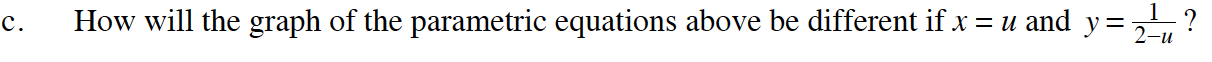### Home > CALC > Chapter 10 > Lesson 10.4.2 > Problem10-155

10-155.
1. Suppose that x = 9t2 and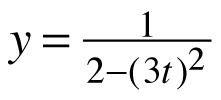for all real t. Homework Help ✎

1. Express x as a function of y.

2. Express y as a function of x.

3. How would the graph of the parametric equations above be different if x = u and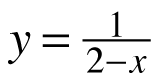for all real u?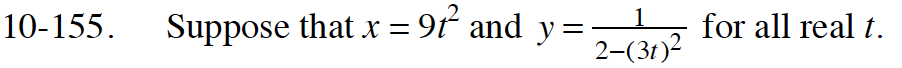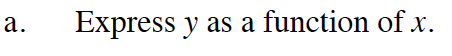Look at the equation for y. (3t)2 = 9t2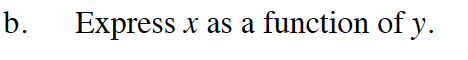Solve your equation from part (a) for x.